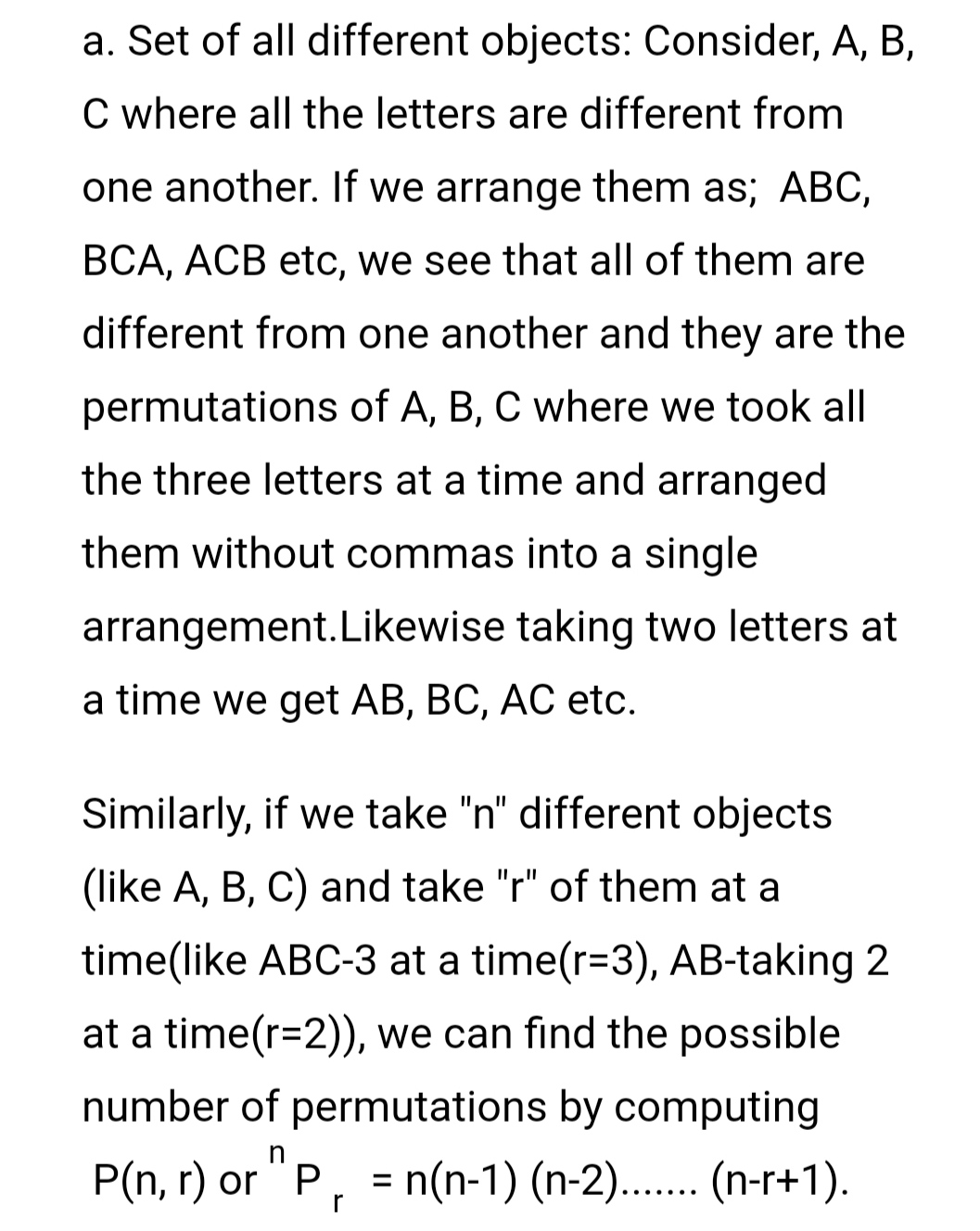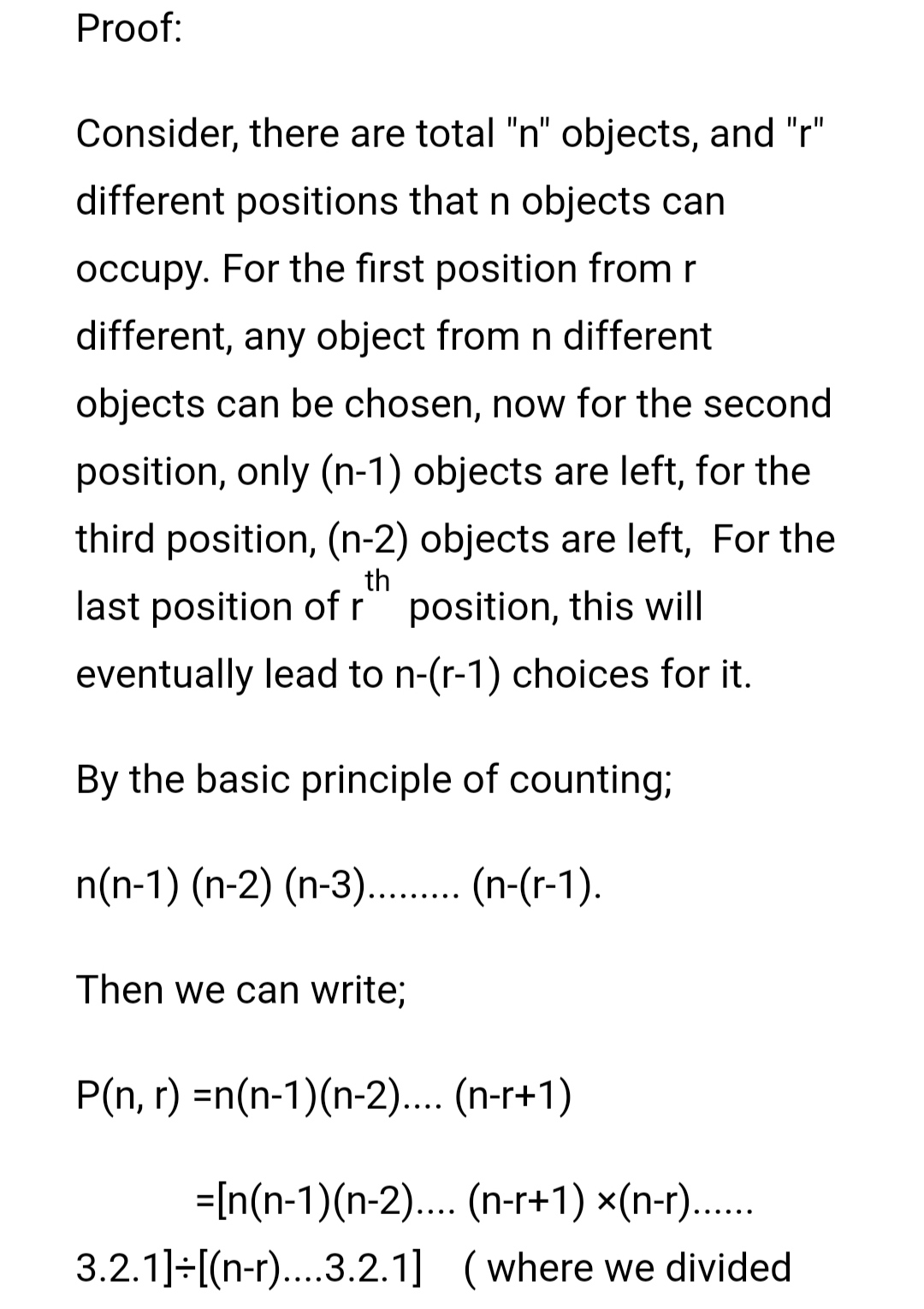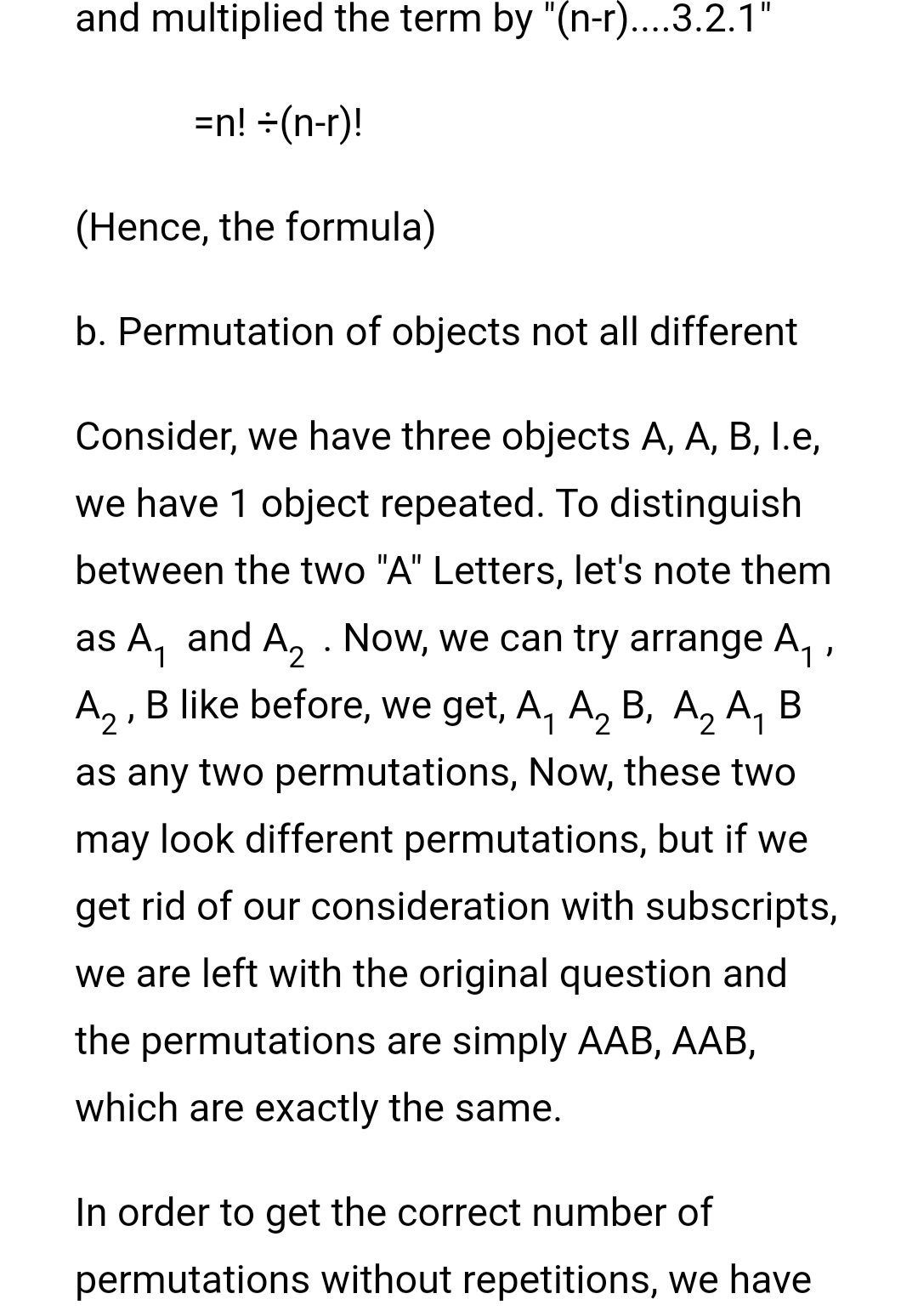# Permutations and Combinations1.Permutationsc. Circular PermutationsGetting the same order only directionally-inversed, they can be regarded as the same and in this case the formula is divided by 2 i.e. 1/2(n-1)!

d. Permutations of repeated things

Consider, n number of objects taken r at a time where each object in n is allowed to repeat itself in r positions, then for the first position we have n different objects while for the second position also, same n objects as the first object can repeat itself, hence the formula becomes;

n×n×n×......... ×r times=nr ways.

2.Combinations

Combination of objects means to combine or collect or include objects where the order in which it is done is not of any significance. The combination of n number if objects taken r at a time is denoted by C(n, r) or  C r .

Let, C(n, r) be a combination where there are r objects which can be arranged among themselves in r! ways i.e r! permutations. So, for each combination in C(n, r), there are C(n, r) r! permutations. Symbolically,

C(n, r). r! = P (n, r) =n! /(n-r)!

C(n, r) =n! /((n-r)! r!)

Clearly, the combinations are divided by the permutations of objects among themselves to exclude out any extra combination due to different order.

Different problems can be identified as the case of permutations or combinations by spotting out the need or needlessness of ordered arrangement in them.

Let us take an example, Suppose, you have school tomorrow with maths, English, science and social classes. The order in which you carry these books with you tomorrow is not of any significance as long as you carry them all (or a fool-proof excuse) with you. Hence, permutations are not important here but only the combinations.

On the other hand, let's say, you have a lockwith you that has a four-digit long password encrypted for you to open it(we all know it's "SHER" but let's give maths a chance) .You have been given four letters A, S, N, T as the letters of the password but simply combining them may not yield out the correct password, here you have to work out alll the possible permutations to find the right order.

Hence, permutations and combinations have revolutionized the provincial counting system with these friendly formulae.